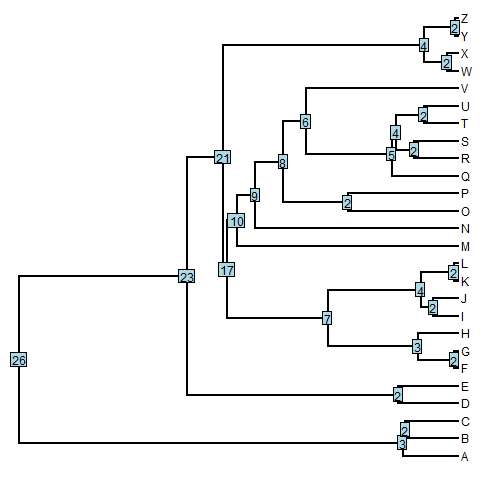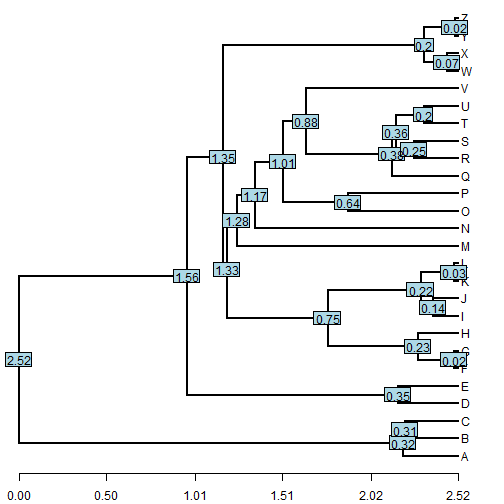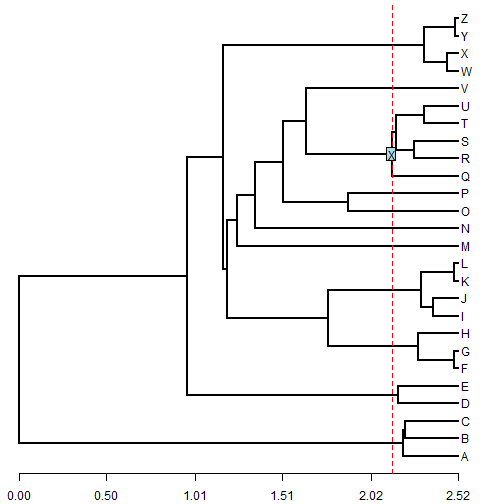## Friday, April 24, 2015

### Finding the youngest node(s) with N or more descendant tips

Today I was asked the following question (slightly paraphrased):

“How do I indentify the node defining the youngest subtree with N (for arbitrary N, in his case it was 5) descendant taxa?”

This is pretty easy. For demonstrative purposes, let's first simulate a tree:

``````library(phytools)
## simulate tree
tree<-pbtree(n=26,tip.label=LETTERS)
``````

Now let's count the number of tips descended from each internal node of the tree:

``````## number of descendant taxa by node
N<-setNames(sapply(1:tree\$Nnode+Ntip(tree),
function(x,tree) sum(getDescendants(tree,x)<=Ntip(tree)),tree=tree),
1:tree\$Nnode+Ntip(tree))
## we can plot them to make sure we got it right
plotTree(tree,mar=c(2.1,0.1,0.1,0.1))
nodelabels(N)
``````Next, we can compute the depth of each node. We can do this in more than one way. Here, I have an `sapply` call around `nodeheight`, but for a larger tree I would use one call to `nodeHeights`.

``````## node depths
d<-setNames(max(nodeHeights(tree))-
sapply(1:tree\$Nnode+Ntip(tree),nodeheight,tree=tree),
1:tree\$Nnode+Ntip(tree))
## again, let's check them by plotting
plotTree(tree,mar=c(2.1,0.1,0.1,0.1))
nodelabels(round(d,2))
axis(1,at=round(0:5/5*max(nodeHeights(tree)),2))
``````Finally, let's find the most recent node (or nodes) with `n=5` or more descendant tips:

``````## which node is the youngest node to have n=5 or more
## descendant taxa
n<-5
node<-as.numeric(names(which(d[N>=n]==min(d[N>=n]))))
``````

Let's check it visually:

``````plotTree(tree,mar=c(2.1,0.1,0.1,0.1))
axis(1,at=round(0:5/5*max(nodeHeights(tree)),2))
ii<-which(names(d)==node)
lines(max(nodeHeights(tree))-c(d[ii],d[ii]),
y=par()\$usr[3:4],lty="dashed",col="red")
nodelabels(node=node,"X")
``````That's it!